# 论文引入

CGAN和CVAE思想是在深度网络下表现较好的两个生成模型，但是除了CVAE和CGAN固有的问题之外，它们的训练对数据集的要求很高，同时一旦数据集的标签 数据增加模型也需要重新训练，这个问题就是上面我们提到的昂贵的成本。如何能在保持条件生成的基础上，解决好上述问题对于条件生成的发展是有很大推动的。 Latent Constraints就是在无监督下训练好生成模型，然后通过改变潜在变量实现有条件的生成，虽然需要带标签的数据集但是可以实现在新增标签数据下 在不重新训练生成模型的前提下实现条件生成。

Latent Constraints的整体思路就是先训练好一个VAE模型，通过有标签信息参与的情况下将先验分布映射到较好的后验分布上，再进一步Decoder出这个 条件下的数据，从而实现无监督模型下有条件生成。总结一下Latent Constraints的优势：

• 实现了从无条件模型中有条件地生成
• 一定程度上解决了VAE生成图像上模糊的问题
• 减小了新增标签数据再训练的计算成本

# 权衡VAE重构和生成

VAE在图像生成上展示了一定的优势，但是由标准VAE生成的图像往往是模糊的，已经有很多研究在改进VAE生成上的模糊但是通过单纯的在VAE基础上改进的话 会发现当控制VAE中的高斯方差$\sigma$的大小以最大化ELBO会造成重构和生成出的样本一个清晰一个很糟。Latent Constraints通过实验找到了当 $\sigma = 0.1$的时候此时VAE的重建效果最好。而我们知道在标准的VAE下$\sigma = 1$，当$\sigma = 0.1$的时候也就是方差变小了，此时整个 高斯分布下采样得到的结果将会更加集中，而将后验分布$q(z \vert x)$通过KL拉向先验分布$p(z)$的话，此时的$p(z)$的采样在表示后验$q(z \vert x)$ 的紧密性上是不充足的，这样就会造成采样生成上的结果很糟糕。但是$\sigma$较小的时候，对于数据的重建效果是好的，我们可以通过实验对比进一步感受一下：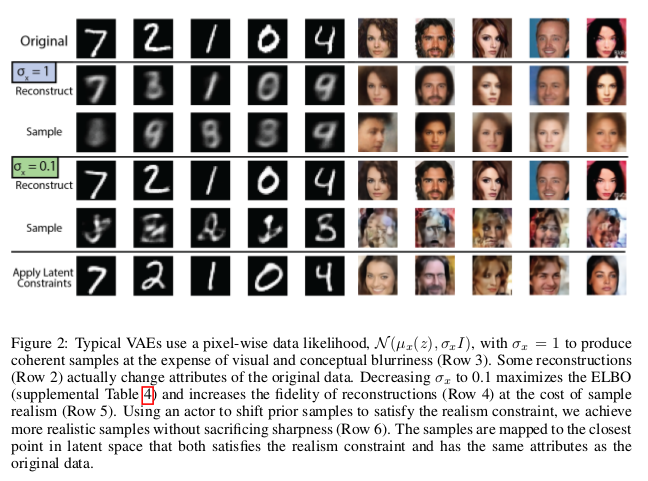# “Realism”判别器

“Realism”判别器的作用就是为了提高在$\sigma = 0.1$的时候采样生成的结果，它的基本原理就是将需要采样的先验分布$p(z)$通过一个生成器G将$p(z)$ 映射到后验分布$q(z \vert x)$得到一个新的潜在变量$z’$，此时的$z’$就可以较好的表示后验分布$q(z \vert x)$，我们知道后验分布$q(z \vert x)$ 做重构出的结果是清晰的，此时在先验分布$p(z)$下做采样经过映射接近$q(z \vert x)$后再生成出的结果应该和重构得到的结果相近，也是清晰的， 这个过程就是“Realism”判别器的目的。

$\mathcal L_{D(z)} = \mathbb E_{z \sim q(z \vert x)}[\mathcal L_{c=1}(z)] + \mathbb E_{z \sim p(z)}[\mathcal L_{c=0}(z)] + \mathbb E_{z \sim G(p(z))}[\mathcal L_{c=0}(z)]$

$\mathcal L_{G(z)} = \mathbb E_{z \sim p(z)}[\mathcal L_{c=1}(G(z)) + \lambda_{dist} \mathcal L_{dist}(G(z),z)]$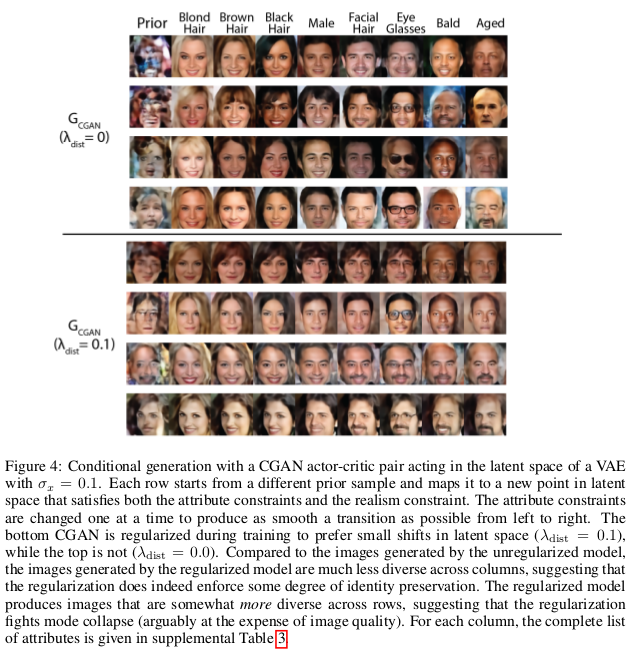# Latent Constraints模型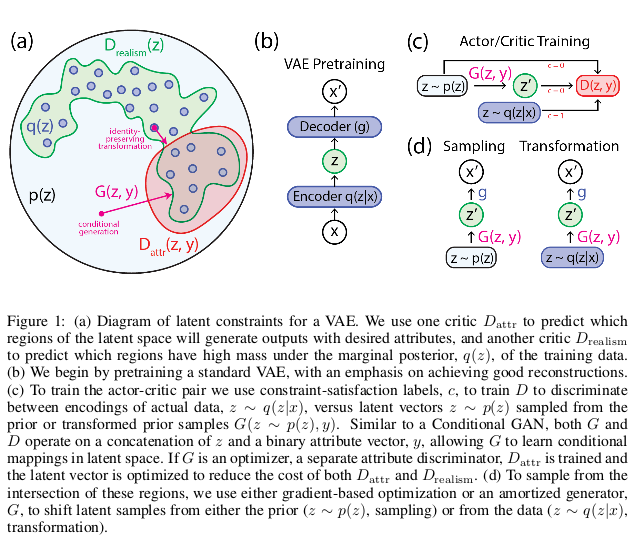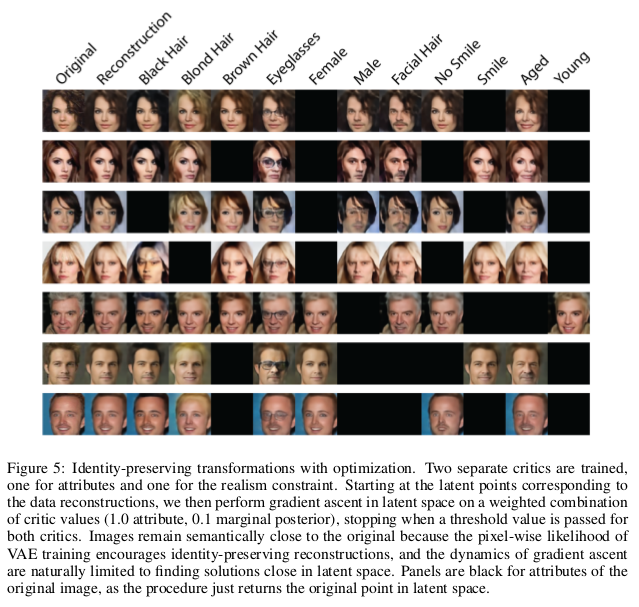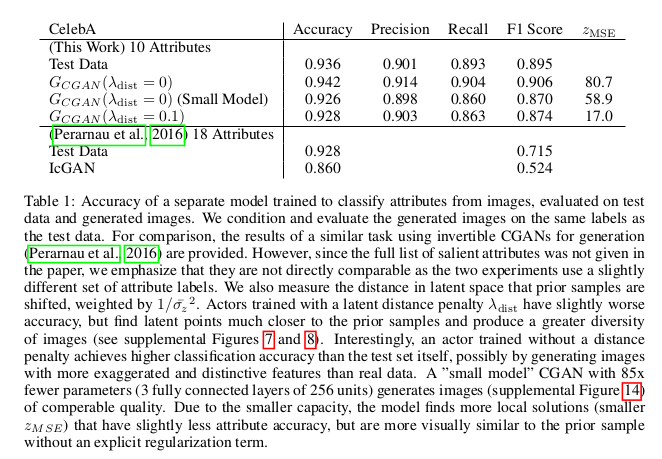# 总结

Latent Constraints通过约束无条件生成模型的潜在空间，展示了一种新的条件生成方法。这种条件生成方法可以大大降低模型在新增标签数据下的训练 成本，有一定的指导意义。同时Latent Constraints也算是将VAE和GAN做了结合，在这一方面也带来了新的启示。